Index

Conventional
Hyperfractionation
Concomitant boost
Options

TDF Calculator is an application for PalmOS handheld computers which implements the linear-quadratic model to compare different radiotherapy fractionation regimens. TDF Calculator and the tutorial below implement the model calculations found in Time, Dose, and Fractionation in Radiotherapy, chapter 22 in Radiobiology for the Radiologist, Fifth Edition, by Eric J. Hall, Lippincott Williams & Wilkins, Philadelphia, 2000, pp 397-418.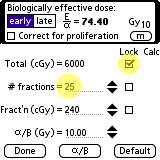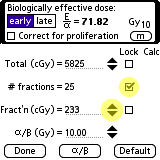The biologically effective dose (E/α) is an approximate quantity by which different fractionation regimens may be intercompared. It is calculated as: E/α = n × d × (1 + (d / (α/β))) where n = number of fractions, d = dose/fraction, total dose = n × d, and α/β = dose at which the linear and quadratic components of cell kill are equal (for early or late effects). Assume a conventional treatment regimen of 30 fractions of 200 cGy given one fraction per day, 5 days per week, for an overall treatment time of 6 weeks. The biologically effective dose (E/α) for early effects is 72 Gy10 and 100 Gy10 for late effects. The subscripts to the Gy are reminders that E/α is not in true grays and of the particular values of α/β used in the calculation. To compare a schedule of 30 fractions with with a new schedule of fewer fractions, you must change the number of fractions in the calculator. You can work with any two of the three inter-related parameters (total dose, n, and d) by locking the parameter you wish to hold constant. Unlocked parameters have editable fields and "up/down" arrow bumpers. You can modify the parameters in predefined increments using the arrow bumpers, or enter exact values using keyboard or Graffiti strokes. E/α is automatically updated in real-time when any input parameter (total dose, n, d or α/β) is changed. Lock the total dose field and change the new number of fractions to 25 as illustrated above. The dose/fraction will change automatically to maintain a total dose of 6000 cGy and the biologically effective dose readout will automatically calculate 74.4 Gy10. Next, relock the number of fractions and reduce the dose/fraction field until the biologically effective dose returns to approximately 72 Gy10. If the assumption of an α/β ratio ≈ 10 Gy is valid, these two schedules should result in approximately equivalent early biological effects. While it is permissible to compare the biologically effective doses calculated for early effects (in Gyn) of one schedule with early effects other schedules (or, likewise, late vs. late effects), it is not permissible or meaningful to compare early with late effects. Switch between early or late effects by tapping the "early" or "late" button next to the E/α readout at the top of the display. The α/β button at the bottom of the calculator displays some data for various tissues from Dr Hall's textbook. There are separate α/β data pages for early and late effects. You can add a few more examples if you wish.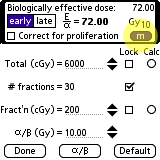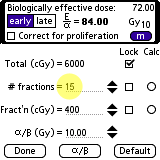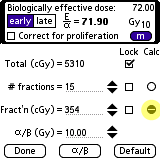An even easier way to calculate the dose per fraction for a new fractionation schedule is to first set the standard schedule and then tap the m (for memory) button to store the current biologically effective dose (BED). The stored BED (72.00 Gy10 in this case) is displayed in the upper right corner. Next, lock the total dose and set the new number of fractions, 15 in this example. Note that the dose/fraction is automatically changed to 400 when you set 15 fractions because the total dose is locked to 6000 cGy. The biologically effective dose for this new schedule of 15 fractions of 400 cGy/fraction is then automatically calculated to be 84.00 Gy10 Now, tap the round Calc button that corresponds to the dose per fraction field. The dose per fraction (354 cGy/frac.) and total dose (5310 cGy) required to match the stored BED of 72.00 Gy10 will be calculated and entered for you. The nearest BED to 72.00 that can be achieved in this example is 71.90 Gy10 because the dose per fraction is constrained by the program to be an integer value.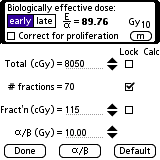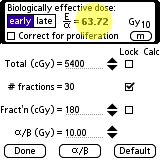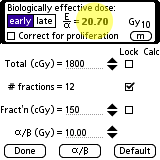This is an example of hyperfractionation. For 70 fractions of 115 cGy given twice daily (BID), 6 hours apart, 5 days per week the biologically effective dose is 89.8 Gy10 for early effects and 111.4 Gy3 for late effects. This treatment is much "hotter," ie more effective than the conventional for both early and late effects. To calculate the biological effectiveness of a concomitant boost of 30 fractions of 180 cGy given once a day, 5 days a week and at the same time (concomitant) a boost to a smaller field of 12 fractions of 150 cGy once a day, you simply add the individual components. The primary component has a biological effectiveness of 63.7 Gy10 for early effects. The boost component has a biological effectiveness of 20.7 Gy10. The concomitant treatment has a biological effectiveness of 63.7 Gy10 + 20.7 Gy10 = 84.4 Gy10 for early effects and 113.4 Gy3 for late effects. The Gyn values should be compared with the comparable values for the alternate schedules. For the example in this tutorial, the concomitant boost is hotter than the conventional schedule for both early and late effects. Compared with hyperfractionation, however, the concomitant boost is almost the same for late effects but less effective for early effects, including tumor control.A correction for tumor proliferation may be added to the calculation if desired. This adds a new term to the model so that the equation for biological effectiveness becomes: E/α = n × d × (1 + (d / (α/β))) - ((0.693 / α) × (t / Tpot)) To include this correction factor tap the "Correct for proliferation" checkbox. where t = time, Tpot = the potential doubling time of the tumor, and α = initial slope of cell survival curve. There is very little data regarding what values to use for α and Tpot, so using this factor is speculative at best.. The biologically effective dose is now 53.98 Gy10 with proliferation included in the calculation compared to 72.00 Gy10 without proliferation.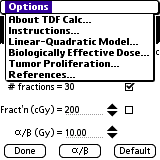Additional options for TDF Calc are accessible by tapping the menu icon in the silkscreen area of the handheld.